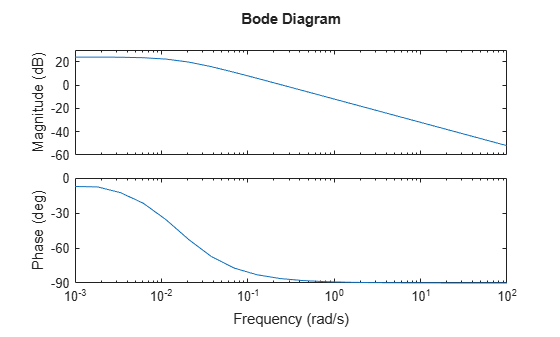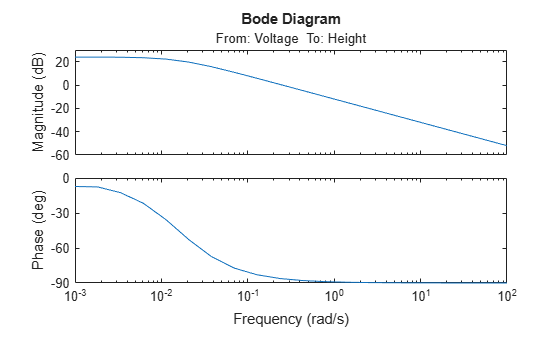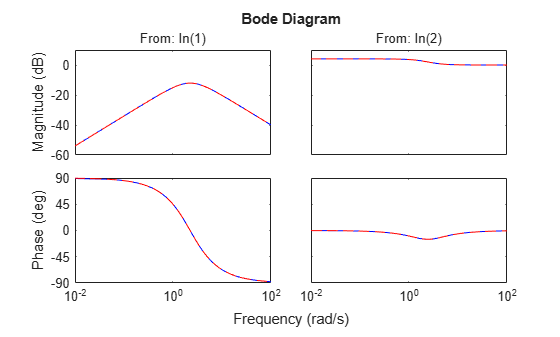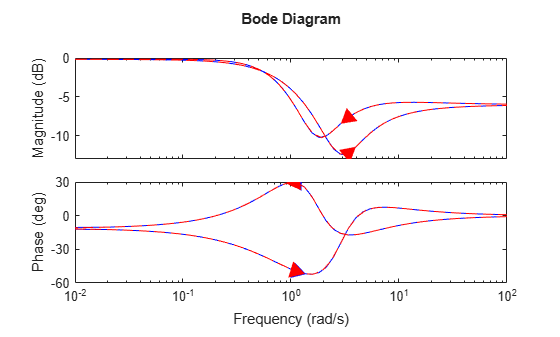# frd

Frequency-response data model

## Description

Use `frd` to create real-valued or complex-valued frequency-response data models, or to convert dynamic system models to frequency-response data model form.

Frequency-response data models store complex frequency response data with corresponding frequency points. For example, a frequency-response data model H(jwi), stores the frequency response at each input frequency wi, where i = 1,…,n. The `frd` model object can represent SISO or MIMO frequency-response data models in continuous time or discrete time. For more information, see Frequency Response Data (FRD) Models.

You can also use `frd` to create generalized frequency-response data (`genfrd`) models.

## Creation

You can obtain `frd` models in one of the following ways.

### Syntax

``sys = frd(response,frequency)``
``sys = frd(response,frequency,ts)``
``sys = frd(response,frequency,ltiSys)``
``sys = frd(___,Name,Value)``
``sys = frd(ltiSys,frequency)``
``sys = frd(ltiSys,frequency,FrequencyUnits)``

### Description

example

````sys = frd(response,frequency)` creates a continuous-time frequency-response data (`frd`) model, setting the `ResponseData` and `Frequency` properties. `frequency` can contain both negative and positive frequencies.```

example

````sys = frd(response,frequency,ts)` creates a discrete-time `frd` model with the sample time `ts`. To leave the sample time unspecified, set `ts` to –1.```

example

````sys = frd(response,frequency,ltiSys)` creates a frequency-response data model with properties inherited from the dynamic system model `ltiSys`, including the sample time.```

example

````sys = frd(___,Name,Value)` sets properties of the frequency-response data model using one or more name-value arguments for any of the previous input-argument combinations.```

example

````sys = frd(ltiSys,frequency)` converts the dynamic system model `ltiSys` to a frequency-response data model. `frd` computes the frequency response at frequencies specified by `frequency`. `sys` inherits its frequency units rad/`TimeUnit` from `ltiSys.TimeUnit`.```
````sys = frd(ltiSys,frequency,FrequencyUnits)` interprets frequencies in the units specified by `FrequencyUnit`.```

### Input Arguments

expand all

Frequency response data, specified as a vector or a multidimensional array of complex numbers.

• For SISO systems, specify a vector of frequency response values at the frequency points specified in `frequency`.

• For MIMO systems with `Nu` inputs and `Ny` outputs, specify a `Ny`-by-`Nu`-by-`Nf` array, where `Nf` is the number of frequency points.

• For an `S1`-...-by-`Sn` array of models with `Nu` inputs and `Ny` outputs, specify a multidimensional array of size [`Ny` `Nu` `Nf` `S1``Sn`].

For instance, a `response` of size [`Ny`,`Nu`,`Nf`,`3`,`4`] represents the response data for a 3-by-4 array of models. Each model has `Ny` outputs, `Nu` inputs, and `Nf` frequency points.

This input sets the ResponseData property.

Frequency points corresponding to `response`, specified as a vector that contains `Nf` points. `frequency` can contain both positive and negative frequencies.

This input sets the Frequency property.

Sample time, specified as a scalar.

The input sets the Ts property.

Dynamic system, specified as a SISO or MIMO dynamic system model or an array of dynamic system models. Dynamic systems that you can use include:

## Properties

expand all

Frequency response data, specified as a multidimensional array of complex numbers.

• For SISO systems, `ResponseData` is a `1`-by-`1`-by-`Nf` array of frequency response values at the `Nf` frequency points specified in the `Frequency` property.

• For MIMO systems with `Nu` inputs and `Ny` outputs, `ResponseData` is an `Ny`-by-`Nu`-by-`Nf` array, where `Nf` is the number of frequency points.

For instance, `ResponseData(ky,ku,kf)` represents the frequency response from the input `ku` to the output `ky` at the frequency `Frequency(kf)`.

• For an `S1`-...-by-`Sn` array of models with `Nu` inputs and `Ny` outputs, `ResponseData` is a multidimensional array of size [`Ny` `Nu` `Nf` `S1``Sn`].

For instance, a `ResponseData` of size [`Ny`,`Nu`,`Nf`,`3`,`4`] represents the response data for a 3-by-4 array of models. Each model has `Ny` outputs, `Nu` inputs, and `Nf` frequency points.

Frequency points corresponding to `ResponseData`, specified as a vector that contains `Nf` points in the units specified by `FrequencyUnit`.

Units of the frequency vector in the `Frequency` property, specified as one of the following values:

• `'rad/TimeUnit'`

• `'cycles/TimeUnit'`

• `'rad/s'`

• `'Hz'`

• `'kHz'`

• `'MHz'`

• `'GHz'`

• `'rpm'`

The units `'rad/TimeUnit'` and `'cycles/TimeUnit'` are relative to the time units specified in the `TimeUnit` property.

Changing this property does not resample or convert the data. Modifying the property changes only the interpretation of the existing data. Use `chgFreqUnit` to convert the data to different frequency units.

Transport delay, specified as one of the following:

• Scalar — Specify the transport delay for a SISO system or the same transport delay for all input/output pairs of a MIMO system.

• `Ny`-by-`Nu` array — Specify separate transport delays for each input/output pair of a MIMO system. Here, `Ny` is the number of outputs and `Nu` is the number of inputs.

For continuous-time systems, specify transport delays in the time unit specified by the `TimeUnit` property. For discrete-time systems, specify transport delays in integer multiples of the sample time, `Ts`.

Input delay for each input channel, specified as one of the following:

• Scalar — Specify the input delay for a SISO system or the same delay for all inputs of a multi-input system.

• `Nu`-by-1 vector — Specify separate input delays for input of a multi-input system, where `Nu` is the number of inputs.

For continuous-time systems, specify input delays in the time unit specified by the `TimeUnit` property. For discrete-time systems, specify input delays in integer multiples of the sample time, `Ts`.

Output delay for each output channel, specified as one of the following:

• Scalar — Specify the output delay for a SISO system or the same delay for all outputs of a multi-output system.

• `Ny`-by-1 vector — Specify separate output delays for output of a multi-output system, where `Ny` is the number of outputs.

For continuous-time systems, specify output delays in the time unit specified by the `TimeUnit` property. For discrete-time systems, specify output delays in integer multiples of the sample time, `Ts`.

Sample time, specified as:

• `0` for continuous-time systems.

• A positive scalar representing the sampling period of a discrete-time system. Specify `Ts` in the time unit specified by the `TimeUnit` property.

• `-1` for a discrete-time system with an unspecified sample time.

Note

Changing `Ts` does not discretize or resample the model.

Time variable units, specified as one of the following:

• `'nanoseconds'`

• `'microseconds'`

• `'milliseconds'`

• `'seconds'`

• `'minutes'`

• `'hours'`

• `'days'`

• `'weeks'`

• `'months'`

• `'years'`

Changing `TimeUnit` has no effect on other properties, but changes the overall system behavior. Use `chgTimeUnit` to convert between time units without modifying system behavior.

Input channel names, specified as one of the following:

• A character vector, for single-input models.

• A cell array of character vectors, for multi-input models.

• `''`, no names specified, for any input channels.

Alternatively, you can assign input names for multi-input models using automatic vector expansion. For example, if `sys` is a two-input model, enter the following:

`sys.InputName = 'controls';`

The input names automatically expand to `{'controls(1)';'controls(2)'}`.

You can use the shorthand notation `u` to refer to the `InputName` property. For example, `sys.u` is equivalent to `sys.InputName`.

Use `InputName` to:

• Identify channels on model display and plots.

• Extract subsystems of MIMO systems.

• Specify connection points when interconnecting models.

Input channel units, specified as one of the following:

• A character vector, for single-input models.

• A cell array of character vectors, for multi-input models.

• `''`, no units specified, for any input channels.

Use `InputUnit` to specify input signal units. `InputUnit` has no effect on system behavior.

Input channel groups, specified as a structure. Use `InputGroup` to assign the input channels of MIMO systems into groups and refer to each group by name. The field names of `InputGroup` are the group names and the field values are the input channels of each group. For example, enter the following to create input groups named `controls` and `noise` that include input channels `1` and `2`, and `3` and `5`, respectively.

```sys.InputGroup.controls = [1 2]; sys.InputGroup.noise = [3 5];```

You can then extract the subsystem from the `controls` inputs to all outputs using the following.

`sys(:,'controls')`

By default, `InputGroup` is a structure with no fields.

Output channel names, specified as one of the following:

• A character vector, for single-output models.

• A cell array of character vectors, for multi-output models.

• `''`, no names specified, for any output channels.

Alternatively, you can assign output names for multi-output models using automatic vector expansion. For example, if `sys` is a two-output model, enter the following.

`sys.OutputName = 'measurements';`

The output names automatically expand to `{'measurements(1)';'measurements(2)'}`.

You can also use the shorthand notation `y` to refer to the `OutputName` property. For example, `sys.y` is equivalent to `sys.OutputName`.

Use `OutputName` to:

• Identify channels on model display and plots.

• Extract subsystems of MIMO systems.

• Specify connection points when interconnecting models.

Output channel units, specified as one of the following:

• A character vector, for single-output models.

• A cell array of character vectors, for multi-output models.

• `''`, no units specified, for any output channels.

Use `OutputUnit` to specify output signal units. `OutputUnit` has no effect on system behavior.

Output channel groups, specified as a structure. Use `OutputGroup`to assign the output channels of MIMO systems into groups and refer to each group by name. The field names of `OutputGroup` are the group names and the field values are the output channels of each group. For example, create output groups named `temperature` and `measurement` that include output channels `1`, and `3` and `5`, respectively.

```sys.OutputGroup.temperature = ; sys.OutputGroup.measurement = [3 5];```

You can then extract the subsystem from all inputs to the `measurement` outputs using the following.

`sys('measurement',:)`

By default, `OutputGroup` is a structure with no fields.

System name, specified as a character vector. For example, `'system_1'`.

User-specified text that you want to associate with the system, specified as a character vector or cell array of character vectors. For example, `'System is MIMO'`.

User-specified data that you want to associate with the system, specified as any MATLAB data type.

Sampling grid for model arrays, specified as a structure array.

Use `SamplingGrid` to track the variable values associated with each model in a model array, including identified linear time-invariant (IDLTI) model arrays.

Set the field names of the structure to the names of the sampling variables. Set the field values to the sampled variable values associated with each model in the array. All sampling variables must be numeric scalars, and all arrays of sampled values must match the dimensions of the model array.

For example, you can create an 11-by-1 array of linear models, `sysarr`, by taking snapshots of a linear time-varying system at times `t = 0:10`. The following code stores the time samples with the linear models.

` sysarr.SamplingGrid = struct('time',0:10)`

Similarly, you can create a 6-by-9 model array, `M`, by independently sampling two variables, `zeta` and `w`. The following code maps the `(zeta,w)` values to `M`.

```[zeta,w] = ndgrid(<6 values of zeta>,<9 values of w>) M.SamplingGrid = struct('zeta',zeta,'w',w)```

When you display `M`, each entry in the array includes the corresponding `zeta` and `w` values.

`M`
```M(:,:,1,1) [zeta=0.3, w=5] = 25 -------------- s^2 + 3 s + 25 M(:,:,2,1) [zeta=0.35, w=5] = 25 ---------------- s^2 + 3.5 s + 25 ...```

For model arrays generated by linearizing a Simulink model at multiple parameter values or operating points, the software populates `SamplingGrid` automatically with the variable values that correspond to each entry in the array. For instance, the Simulink Control Design commands `linearize` (Simulink Control Design) and `slLinearizer` (Simulink Control Design) populate `SamplingGrid` automatically.

By default, `SamplingGrid` is a structure with no fields.

## Object Functions

The following lists contain a representative subset of the functions you can use with `frd` models. In general, many functions applicable to Dynamic System Models are also applicable to a `frd` object. `frd` models do not work with any time-domain analysis functions.

expand all

 `bode` Bode plot of frequency response, or magnitude and phase data `sigma` Singular value plot of dynamic system `nyquist` Nyquist plot of frequency response `nichols` Nichols chart of frequency response `bandwidth` Frequency response bandwidth `freqresp` Evaluate system response over a grid of frequencies `margin` Gain margin, phase margin, and crossover frequencies
 `chgFreqUnit` Change frequency units of frequency-response data model `chgTimeUnit` Change time units of dynamic system `frdfun` Apply a function to the frequency response value at each frequency of an `frd` model object `fselect` Select frequency points or range in FRD model `interp` Interpolate FRD model `fcat` Concatenate FRD models along frequency dimension `fnorm` Pointwise peak gain of FRD model
 `feedback` Feedback connection of multiple models `connect` Block diagram interconnections of dynamic systems `series` Series connection of two models `parallel` Parallel connection of two models
 `pidtune` PID tuning algorithm for linear plant model

## Examples

collapse all

Create an `frd` object from frequency response data.

For this example, load the frequency response data collected for a water tank model.

`load wtankData.mat`

This data contains the frequency response data collected for the frequency range ${10}^{-3}$ rad/s to ${10}^{2}$ rad/s.

Create the model.

`sys = frd(response,frequency)`
```sys = Frequency(rad/s) Response ---------------- -------- 0.0010 1.562e+01 - 1.9904i 0.0018 1.560e+01 - 2.0947i 0.0034 1.513e+01 - 3.3670i 0.0062 1.373e+01 - 5.4306i 0.0113 1.047e+01 - 7.5227i 0.0207 5.829e+00 - 7.6529i 0.0379 2.340e+00 - 5.6271i 0.0695 7.765e-01 - 3.4188i 0.1274 2.394e-01 - 1.9295i 0.2336 7.216e-02 - 1.0648i 0.4281 2.157e-02 - 0.5834i 0.7848 6.433e-03 - 0.3188i 1.4384 1.916e-03 - 0.1740i 2.6367 5.705e-04 - 0.0950i 4.8329 1.698e-04 - 0.0518i 8.8587 5.055e-05 - 0.0283i 16.2378 1.505e-05 - 0.0154i 29.7635 4.478e-06 - 0.0084i 54.5559 1.333e-06 - 0.0046i 100.0000 3.967e-07 - 0.0025i Continuous-time frequency response. ```

Plot `sys`.

`bode(sys)`For this example, consider randomly generated response data and frequencies.

Generate a 3-by-2-by-7 complex array and a frequency vector with seven points between 0.01 and 100 rad/s. Set the sample time `Ts` to 5 seconds.

```rng(0) r = randn(3,2,7)+1i*randn(3,2,7); w = logspace(-2,2,7); Ts = 5;```

Create the model.

`sys = frd(r,w,Ts)`
```sys = From input 1 to: Frequency(rad/s) output 1 output 2 output 3 ---------------- -------- -------- -------- 0.0100 0.5377 + 0.3192i 1.8339 + 0.3129i -2.2588 - 0.8649i 0.0464 -0.4336 + 1.0933i 0.3426 + 1.1093i 3.5784 - 0.8637i 0.2154 0.7254 - 0.0068i -0.0631 + 1.5326i 0.7147 - 0.7697i 1.0000 1.4090 - 1.0891i 1.4172 + 0.0326i 0.6715 + 0.5525i 4.6416 0.4889 - 1.4916i 1.0347 - 0.7423i 0.7269 - 1.0616i 21.5443 0.8884 - 0.1924i -1.1471 + 0.8886i -1.0689 - 0.7648i 100.0000 0.3252 - 0.1774i -0.7549 - 0.1961i 1.3703 + 1.4193i From input 2 to: Frequency(rad/s) output 1 output 2 output 3 ---------------- -------- -------- -------- 0.0100 0.8622 - 0.0301i 0.3188 - 0.1649i -1.3077 + 0.6277i 0.0464 2.7694 + 0.0774i -1.3499 - 1.2141i 3.0349 - 1.1135i 0.2154 -0.2050 + 0.3714i -0.1241 - 0.2256i 1.4897 + 1.1174i 1.0000 -1.2075 + 1.1006i 0.7172 + 1.5442i 1.6302 + 0.0859i 4.6416 -0.3034 + 2.3505i 0.2939 - 0.6156i -0.7873 + 0.7481i 21.5443 -0.8095 - 1.4023i -2.9443 - 1.4224i 1.4384 + 0.4882i 100.0000 -1.7115 + 0.2916i -0.1022 + 0.1978i -0.2414 + 1.5877i Sample time: 5 seconds Discrete-time frequency response. ```

The specified data results in a two-input, three-output `frd` model.

For this example, create a frequency-response data model with properties inherited from a transfer function model.

Create a transfer function `sys1` with the `TimeUnit` property set to `'minutes'` and `InputDelay` property set to 3.

```numerator1 = [2,0]; denominator1 = [1,8,0]; sys1 = tf(numerator1,denominator1,'TimeUnit','minutes','InputDelay',3)```
```sys1 = 2 s exp(-3*s) * --------- s^2 + 8 s Continuous-time transfer function. ```
`propValues1 = {sys1.TimeUnit,sys1.InputDelay}`
```propValues1=1×2 cell array {'minutes'} {} ```

Create an `frd` model with properties inherited from `sys1`.

```rng(0) response = randn(1,1,7)+1i*randn(1,1,7); w = logspace(-2,2,7); sys2 = frd(response,w,sys1)```
```sys2 = Frequency(rad/minute) Response --------------------- -------- 0.0100 0.5377 + 0.3426i 0.0464 1.8339 + 3.5784i 0.2154 -2.2588 + 2.7694i 1.0000 0.8622 - 1.3499i 4.6416 0.3188 + 3.0349i 21.5443 -1.3077 + 0.7254i 100.0000 -0.4336 - 0.0631i Input delays (minutes): 3 Continuous-time frequency response. ```
`propValues2 = {sys2.TimeUnit,sys2.InputDelay}`
```propValues2=1×2 cell array {'minutes'} {} ```

Observe that the `frd` model `sys2` has that same properties as `sys1`.

For this example, load the frequency response data collected for a water tank model.

`load wtankData.mat`

The model has one input, Voltage, and one output, Water height.

Create an `frd` model, specifying the input and output names.

`sys = frd(response,frequency,'InputName','Voltage','OutputName','Height');`

Plot the frequency response.

`bode(sys)`The input and output names appear on the Bode plot. Naming the inputs and outputs can be useful when dealing with response plots for MIMO systems.

For this example, compute the `frd` model of the following state-space model:

`$\mathit{A}=\left[\begin{array}{cc}-2& -1\\ 1& -2\end{array}\right],\text{\hspace{0.17em}}\mathit{B}=\left[\begin{array}{cc}1& 1\\ 2& -1\end{array}\right],\text{\hspace{0.17em}}\mathit{C}=\left[\begin{array}{cc}1& 0\end{array}\right],\text{\hspace{0.17em}}\mathit{D}=\left[\begin{array}{cc}0& 1\end{array}\right]\text{\hspace{0.17em}}$`

Create a state-space model using the state-space matrices.

```A = [-2 -1;1 -2]; B = [1 1;2 -1]; C = [1 0]; D = [0 1]; ltiSys = ss(A,B,C,D);```

Convert the state-space model `ltiSys` to a `frd` model for frequencies between 0.01 and 100 rad/s.

```w = logspace(-2,2,50); sys = frd(ltiSys,w);```

Compare the frequency responses.

`bode(ltiSys,'b',sys,'r--')`The responses are identical.

To create arrays of `frd` models, you can specify a multidimensional array of frequency response data.

For instance, when you specify the response data as a numeric array of size [`NY` `NU` `NF` `S1` ... `Sn`], the function returns a `S1`-by-...-by-`Sn` array of `frd` models. Each of these models has `NY` outputs, `NU` inputs, and `NF` frequency points.

Generate a 2-by-3 array of random response data with one-output, two-input models at 10 frequency points between 0.1 and 10 rad/s.

```w = logspace(-1,1,10); r = randn(1,2,10,2,3)+1i*randn(1,2,10,2,3); sys = frd(r,w);```

Extract the model at the index (2,1) from the model array.

`sys21 = sys(:,:,2,1)`
```sys21 = From input 1 to: Frequency(rad/s) output 1 ---------------- -------- 0.1000 0.6715 + 0.0229i 0.1668 0.7172 - 1.7502i 0.2783 0.4889 - 0.8314i 0.4642 0.7269 - 1.1564i 0.7743 0.2939 - 2.0026i 1.2915 0.8884 + 0.5201i 2.1544 -1.0689 - 0.0348i 3.5938 -2.9443 + 1.0187i 5.9948 0.3252 - 0.7145i 10.0000 1.3703 - 0.2248i From input 2 to: Frequency(rad/s) output 1 ---------------- -------- 0.1000 -1.2075 - 0.2620i 0.1668 1.6302 - 0.2857i 0.2783 1.0347 - 0.9792i 0.4642 -0.3034 - 0.5336i 0.7743 -0.7873 + 0.9642i 1.2915 -1.1471 - 0.0200i 2.1544 -0.8095 - 0.7982i 3.5938 1.4384 - 0.1332i 5.9948 -0.7549 + 1.3514i 10.0000 -1.7115 - 0.5890i Continuous-time frequency response. ```

You can specify negative frequency values in an frd object. This capability is useful when you want to capture the frequency response data of models with complex coefficients.

Create a frequency vector with both positive and negative values.

`w0 = sort([-logspace(-2,2,50) 0 logspace(-2,2,50)]);`

Create a state-space model with complex coefficients.

```A = [-3.50,-1.25-0.25i;2,0]; B = [1;0]; C = [-0.75-0.5i,0.625-0.125i]; D = 0.5; Gc = ss(A,B,C,D);```

Convert the model to an frd model at the specified frequencies.

`sys = frd(Gc,w0);`

Plot the frequency response of the models.

`bode(Gc,'b',sys,'r--')`The plot responses match closely. The plot shows two branches for models with complex coefficients, one for positive frequencies, with a right-pointing arrow, and one for negative frequencies, with a left-pointing arrow. In both branches, the arrows indicate the direction of increasing frequencies.

## Version History

Introduced before R2006a